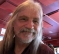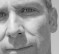## Block Floating Point in FFT.Started by 2 years ago15 replieslatest reply 2 years ago253 views

Hi.

I've seen other post like this on the forum, but it is still not clear to me so I will ask. Sorry if it seems like a repost.

In order to handle the bit growth in the FFT/IFFT algorithm I'm increasing the bit width by one each butterfly stage. This of course increases the gate count which is unwanted as I want to make everything as compact as possible.

After researching, a suggested solution seems to be to scale (shift right) the result after each butterfly stage if necessary. This is called Block Floating Point. Here comes what I don't understand:

If a scaling is to be done after a butterfly it must also be done on all the other samples. However, in an pipeline FFT structure samples may have already "left" the FFT, and can therefore not be scaled. A solution is to include a memory, but this beats the whole purpose of making a more compact FFT.

Best regards, Matias

[ - ]If you wanna design this block-floating-point FFT from scratch, I would recommend that you find yourself a manual for the old Motorola DSP56002 or later (the 56000 and 56001 do not have this feature).  They had a sticky growth bit in their condition-code register for the purpose of block-floating-point FFT.

You start out with all of your input data scaled to have at least 12 dB of headroom.  That is no sample exceeds 1/4 full scale in magnitude of either the real or imaginary parts.  That means the 3 most significant bits must be 000xxxxx or 111xxxxx

Then your FFT must have two different optimized butterflies, one in which the output is scaled by a factor of 1/2, another butterfly in which the output is not scaled by a factor of 1/2.

Now, before an FFT pass begins, you decide which of those scaling modes you will use for the entire pass.  You must not switch the scaling mode midway through a pass, but only between passes.  You start out each pass with the sticky growth bit set to 0.  If, in *any* butterfly result, either the real or the imaginary parts of the butterfly result exceeds 1/4 full scale in magnitude (that is the most significant 3 bits are any other than 000 or 111), then you set the sticky growth bit to 1.  That sticky bit remains set to 1 until you explicitly clear the bit between passes.

If, at the end of a pass, the sticky bit is 1, that means your data has somewhere exceeded the -12 dB ceiling and the following FFT pass should be in the scale-by-1/2 mode.  If the sticky bit is 0, that means all of your FFT bins have data that has not exceeded your -12 dB ceiling and the following FFT pass should have normal scaling (not scaled by 1/2).

Also count how many FFT passes were under the scale-by-1/2 mode and adjust the exponent for your block-floating-point by that amount.

[ - ]Thank you for a very thorough reply. I now have the Motorola DSP56002.

Your explanation helped me clarify some misunderstandings I have had. Like that you have to do the scaling for the entire pass.

Just to be sure, when you say "pass" this means when a butterfly operation has been done on every input sample. I.e. a complete stage in a butterfly diagram has been computed? If so, this would refer to a none pipelined structure, am I right?

When reading a bit through Xilinx FFT IP I found the text (p.13):

- When block floating-point is selected for the Pipelined Streaming I/O architecture, a RAM buffer is required for natural order and bit reversed order output data.

Which implies that you would need extra RAM to use block floating-point in a pipelined design. Just a note to my own question.

[ - ]yes, the term "pass" means processing the entire FFT array once.  if $$N = 2^p$$ is the size of the FFT, then $$p = \log_2(N)$$ is the number passes of the radix-2 FFT.

because the "reach" or "stride" of the FFT butterfly varies from 2 up to half of the size of the FFT, i don't see any way to avoid processing each pass in an array of memory.

if you are processing data in the frequency domain and inverse FFTing back to the time domain, then i might consider a forward FFT based on Decimation-in-Frequency with normal order in and bit-reversed order out, and another inverse FFT based on Decimation-in-Time, with bit-reversed order in and normal order out.  both are In-Place computation so you need only one memory array.  i dunno about pipelining, but for a given In-Place FFT, you can do as many of the butterflies in parallel as you can afford to commit gates in your chip.  the butterflies are all independent.

also, you might want to consider a radix-4 FFT that accomplishes two passes in one with a 4-in and 4-out superbutterfly.  but then bit growth could be more than one bit per pass.  it could be two bits per pass.  i think a simple radix-2 FFT would be simpler.  especially if you'll do block floating point.

[ - ]You cannot shift any single butterfly left you need to do the whole stage, for this reason you might want to allocate yourself a bit of headroom and if at the end of the stage it the headroom in use then on the next stage shift all the butterflies down by one.

[ - ]You actually want two bits of headroom, not just one.  you could have maxxed both your real and imaginary parts, and then have that turned 45 degrees by the FFT twiddle factor which will make one of the real or imaginary part scaled up by $$\sqrt{2}$$.  And that can be added to another number at the max, so things can get scaled by as much as $$1+\sqrt{2} > 2$$.  So you need more than one bit (or 6 dB) of headroom.  You should have two bits or 12 dB of headroom.

[ - ]Post deleted by author

[ - ]Hi Robert, must be very frustrating for you - really sorry.

Here's the html code of your post:

<p>You actually want <strong>two</strong> bits of headroom, not just one.  you could have maxxed both your real and imaginary parts, and then have that turned 45 degrees by the FFT twiddle factor which will make one of the real or imaginary part scaled up by $\sqrt{2}$.  And that can be added to another number at the max, so things can get scaled by as much as $1+\sqrt{2} > 2$.  So you need more than one bit (or 6 dB) of headroom.  You should have two bits or 12 dB of headroom.</p>

The first thing that I notice is that you are not using the right delimiters for inline code. A long time ago, I switched the settings from using the single dollar sign to:



$$\sqrt{2}$$


should work: $$\sqrt{2}$$

And for your second equation, there is a

&gt;


in your equation, which is the html code for '>'.  I am not sure how it ended up there, maybe it has something to do with the origin of your copy/paste, but I would suggest to edit your html code before posting and correct annoyances like that.

$$1+\sqrt{2} > 2$$


Should also work: $$1+\sqrt{2} > 2$$

[ - ]Post deleted by author

[ - ]It is two dollar signs for equations that will have their own line (as opposed to 'in-line'). So:

$$\sqrt{2}$$


Would result in:

$$\sqrt{2}$$

May I suggest you check out the 'pinned' post at the top of the forum as a quick reminder when you need to add an equation to your posts?  In the meantime, I will certainly keep in mind that the current way of doing equations is not very intuitive and should be improved.

[ - ]Stephane, it's too late i know, but when you were implementing mathjax or whatever mechanism to render $$\LaTeX$$ math, i would have picked an existing protocol for it.

The DSP Stack exchange uses single and double dollar signs.  So also do the math and physics SE sites.

The EE stack exchange uses double dollar for stand-alone, but, i presume this was the same issue for you, that in an engineering post, cost and money is part of the discussion so the single dollar sign was not used for a delimiter, but rather than introduce the parenth, they just do "\\$" to delimit in-line math.

Wikipedia uses "$" and "$".

So now i have to remember four different protocols.  my 64 year old brain cells just can't do it.

and using Tex is a whole 'nother bag.

[ - ]Thank you for pointing that out, I just read about this and I understand it better now. Is it correct that you would only need to consider the scaling of $$1+\sqrt{2}$$ at the first butterfly stage/pass though? Since after, the maximum imaginary and real part lies on a circle with radius $$1+\sqrt{2}$$ , and a multiplication with the twiddle factor will only rotate the sample around that circle.

[ - ]If I understand it correctly, this mean that I need to compute all the samples for each butterfly stage before sending them to the next stage. But then I won't be able to use a pipelined structure, or?

[ - ]There are many ways to deal with this but one would be to have the butterfly operator include an optional pre-left-shift. Each butterfly would also update a register indicating the amount of headroom in the output of its butterfly. After a stage has completed the stages headroom could be found then for the next stage if there was 0 bits of headroom in the output of the last then the optional pre-left-shift would be enabled.

[ - ]